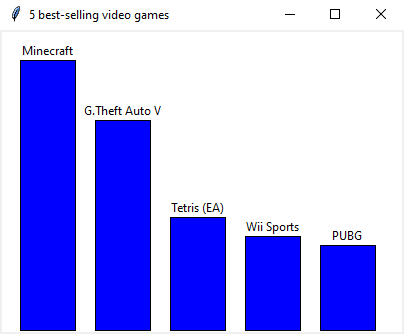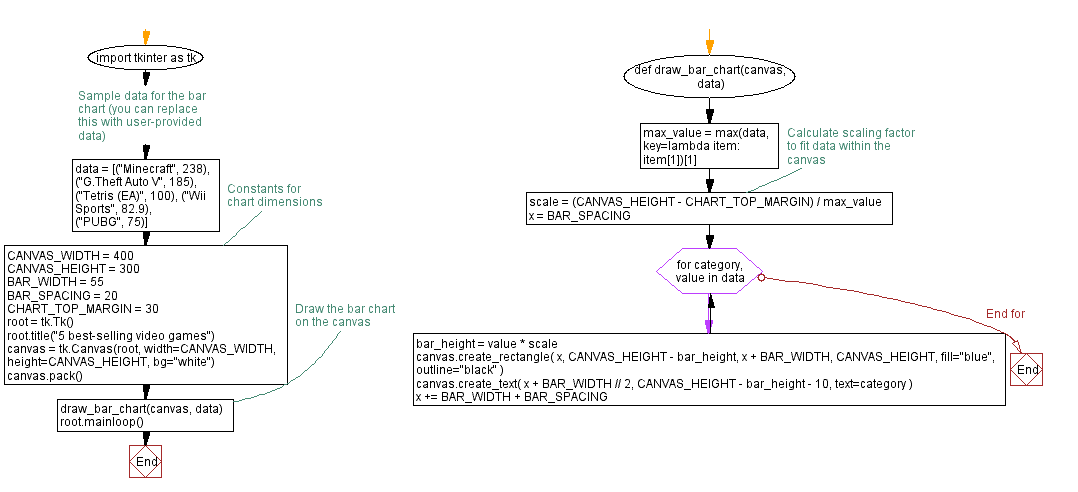﻿ Create interactive charts with Python and Tkinter

# Build interactive charts and graphs with Python and Tkinter

## Python Tkinter Canvas and Graphics: Exercise-12 with Solution

Write a Python program that develops a program that uses the Canvas widget to draw a chart or graph (e.g., bar chart, pie chart) based on user-provided data using Tkinter.

Sample Solution:

Python Code:

``````import tkinter as tk

# Sample data for the bar chart (you can replace this with user-provided data)
data = [("Minecraft", 238), ("G.Theft Auto V", 185), ("Tetris (EA)", 100), ("Wii Sports", 82.9), ("PUBG", 75)]

# Constants for chart dimensions
CANVAS_WIDTH = 400
CANVAS_HEIGHT = 300
BAR_WIDTH = 55
BAR_SPACING = 20
CHART_TOP_MARGIN = 30

def draw_bar_chart(canvas, data):
max_value = max(data, key=lambda item: item)

# Calculate scaling factor to fit data within the canvas
scale = (CANVAS_HEIGHT - CHART_TOP_MARGIN) / max_value

x = BAR_SPACING

for category, value in data:
bar_height = value * scale
canvas.create_rectangle(
x, CANVAS_HEIGHT - bar_height, x + BAR_WIDTH, CANVAS_HEIGHT,
fill="blue", outline="black"
)
canvas.create_text(
x + BAR_WIDTH // 2, CANVAS_HEIGHT - bar_height - 10, text=category
)
x += BAR_WIDTH + BAR_SPACING

root = tk.Tk()
root.title("5 best-selling video games")

canvas = tk.Canvas(root, width=CANVAS_WIDTH, height=CANVAS_HEIGHT, bg="white")
canvas.pack()

# Draw the bar chart on the canvas
draw_bar_chart(canvas, data)

root.mainloop()
```
```

Explanation:

In the exercise above -

• Define sample data in the data list.
• Specify constants for chart dimensions, such as canvas width and height, bar width, bar spacing, and chart top margin.
• The "draw_bar_chart()" function takes the canvas and data as arguments and draws the bar chart on the canvas. In order to fit the chart within the canvas, it calculates a scaling factor based on the maximum value in the data.
• For each data point, draw rectangles (bars) on the canvas inside the function. The height of each bar is determined by the value scaled by the scaling factor.
• Label each bar with the corresponding category.
• Finally, create the Tkinter main window, create a canvas widget, and call the "draw_bar_chart()" function to draw the bar chart on the canvas.

Output:

``````

Flowchart:Python Code Editor:

What is the difficulty level of this exercise?

Test your Programming skills with w3resource's quiz.

﻿

## Python: Tips of the Day

Summing a sequence of numbers (calculating the sum of zero to ten with skips):

```>>> l = range(0,10,2)
>>> sum(l)
20
```

We are closing our Disqus commenting system for some maintenanace issues. You may write to us at reach[at]yahoo[dot]com or visit us at Facebook